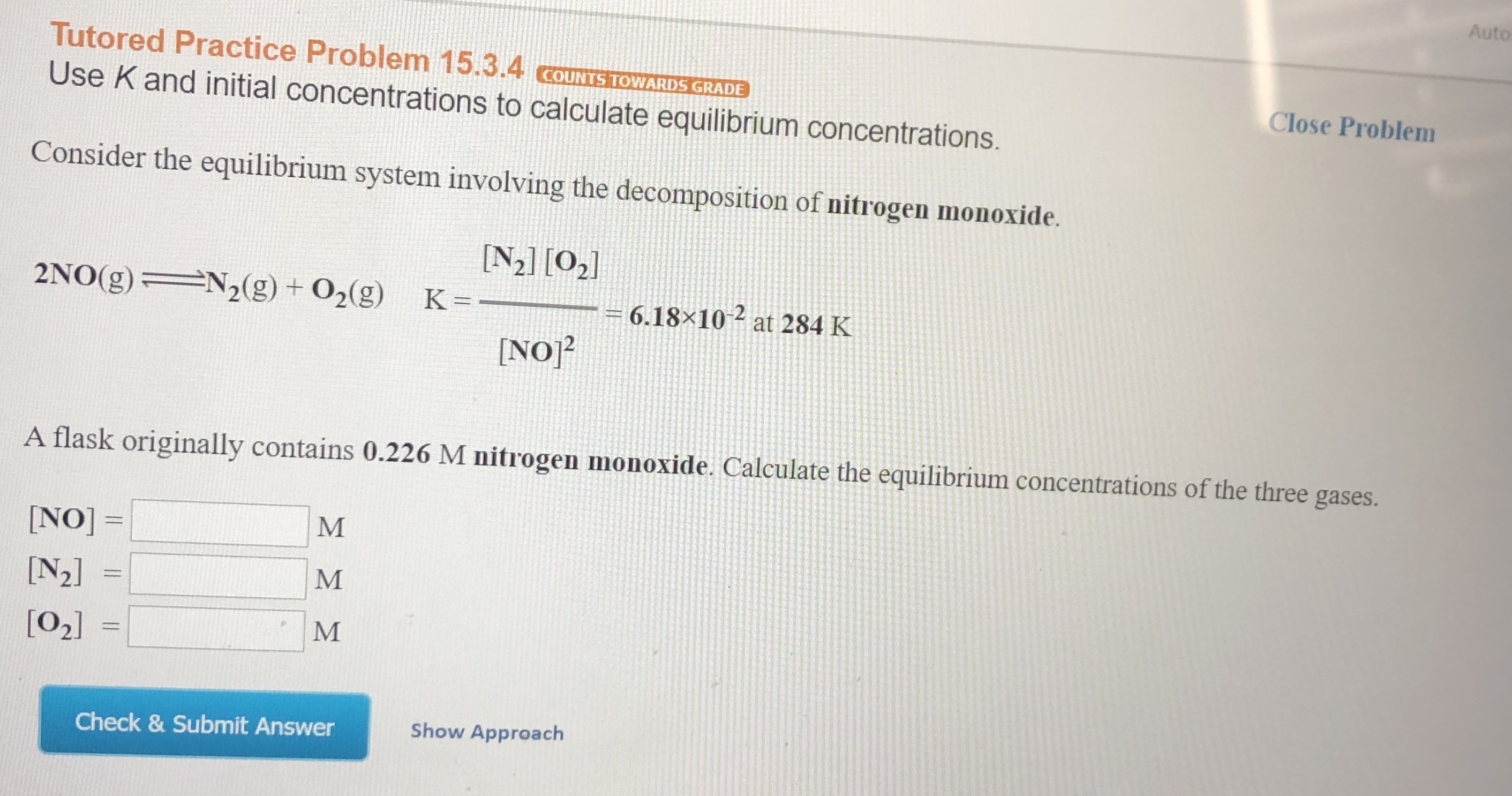# Auto Tutored Practice Problem 15.3.4 cOUNTS TOWARDS GRADE Close Problem Use K and initial concentrations to calculate equilibrium concentrations. Consider the equilibrium system involving the decomposition of nitrogen monoxide. N2] [02 N2(g) + 02(g) 2NO(g) K = 6.18x10 at 284 K [NO2 A flask originally contains 0.226 M nitrogen monoxide. Calculate the equilibrium concentrations of the three gases. M NO]= [N2] M  Show Approach Check & Submit Answer

Questionhelp_outlineImage TranscriptioncloseAuto Tutored Practice Problem 15.3.4 cOUNTS TOWARDS GRADE Close Problem Use K and initial concentrations to calculate equilibrium concentrations. Consider the equilibrium system involving the decomposition of nitrogen monoxide. N2] [02 N2(g) + 02(g) 2NO(g) K = 6.18x10 at 284 K [NO2 A flask originally contains 0.226 M nitrogen monoxide. Calculate the equilibrium concentrations of the three gases. M NO]= [N2] M  Show Approach Check & Submit Answer fullscreen

### Want to see this answer and more?

Experts are waiting 24/7 to provide step-by-step solutions in as fast as 30 minutes!*

*Response times vary by subject and question complexity. Median response time is 34 minutes and may be longer for new subjects.
Tagged in
ScienceChemistry

### General Chemistry